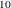### ODS Graphics

Statistical procedures use ODS Graphics to create graphs as part of their output. ODS Graphics is described in detail in Chapter 21: Statistical Graphics Using ODS.

Before you create graphs, ODS Graphics must be enabled (for example, by specifying the ODS GRAPHICS ON statement). For more information about enabling and disabling ODS Graphics, see the section Enabling and Disabling ODS Graphics in Chapter 21: Statistical Graphics Using ODS.

The overall appearance of graphs is controlled by ODS styles. Styles and other aspects of using ODS Graphics are discussed in the section A Primer on ODS Statistical Graphics in Chapter 21: Statistical Graphics Using ODS.

You must also specify the options in the PROC MULTTEST statement that are indicated in Table 61.4.

PROC MULTTEST assigns a name to each graph it creates using ODS. You can use these names to reference the graphs when using ODS. The names are listed in Table 61.4.

Table 61.4: Graphs Produced by PROC MULTTEST

ODS Graph Name

Plot Description

Option

Adjusted by rank of raw p-values

FalsePosBySignificant

Expected number of false positives by proportion significant

p-values by test

PLOTS=PBYTEST

MSE or NTRUENULL by lambda

PLOTS=LAMBDA and
(NTRUENULL=BOOTSTRAP
or NTRUENULL=SPLINE
or PFDR)

–log(adjusted p-values) by test

PLOTS=MANHATTAN

ManhattanPanel

Panel of Manhattan plots

PLOTS=MANHATTAN

Raw p-values by rank and histogram

PLOTS=RAWPROB or
AHOLM or AHOC or AFDR or PFDR

RawUniformPlot

Raw p-values by rank

PLOTS=RAWPROB(UNPACK) and
AHOLM or AHOC or AFDR or PFDR

RawUniformHist

Histogram of raw p-values

PLOTS=RAWPROB(UNPACK) and
AHOLM or AHOC or AFDR or PFDR